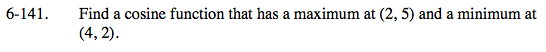### Home > PC > Chapter 6 > Lesson 6.4.1 > Problem6-141

6-141.Find a cosine function that has a maximum at (2, 5) and a minimum at (4, 2). Homework Help ✎$\text{amplitude}=\frac{\text{max }-\text{ min}}{2}$

Vertical shift: Since the minimum point is at y = 2 and the amplitude is 1.5, the vertical shift would be their sum.

Horizontal shift: Since the maximum point is at (2, 5) a cosine function has been shifted 2 units right.

Since a maximum is at x = 2 and a minimum is at x = 4, half of a cycle is 2 units. Hence, the period is 4.
Use the formula pb = 2π to calculate b.